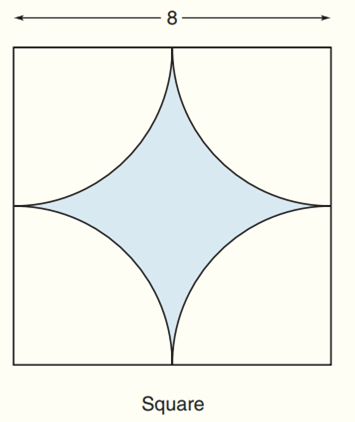Chapter 8.CR, Problem 27CR### Elementary Geometry for College St...

6th Edition
Daniel C. Alexander + 1 other
ISBN: 9781285195698

#### Solutions

Chapter
Section### Elementary Geometry for College St...

6th Edition
Daniel C. Alexander + 1 other
ISBN: 9781285195698
Textbook Problem
1 views

# Find the exact areas of the shaded regions in Exercises 27 to 31.To determine

To find:

The exact areas of the shaded regions.

Explanation

Theorem:

The area A of a square whose sides are each of length s is given by A=s2

Calculation:

Sketch the following diagram:

The side length (s) = 8

The area of quarter circle = 14πr2

The area of shaded region = Area of square - 4×area of quarter circle=

### Still sussing out bartleby?

Check out a sample textbook solution.

See a sample solution

#### The Solution to Your Study Problems

Bartleby provides explanations to thousands of textbook problems written by our experts, many with advanced degrees!

Get Started

#### Find more solutions based on key concepts